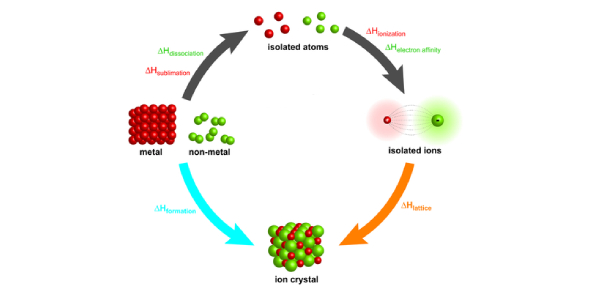# Born Haber Cycle MCQ Quiz With Answers

10 Questions | Attempts: 2706
ShareSettingsDo you know about lattice energy and Born Haber Cycle? Can you attempt this MCQ quiz on lattice energy and Born Haber Cycle? Give this quiz a try and check your knowledge of these two concepts. The Born–Haber cycle is a method for analyzing energies of chemical reactions, and lattice energy can be calculated using this cycle. You will be asked to calculate lattice energies for the given compounds. Will you be able to do so? Let's check it out with this lattice energy quiz.

• 1.
Which of the following compounds is expected to have the highest lattice energy?
• A.

NACl

• B.

NaBr

• C.

MgF2

• D.

All of these

• 2.
Consider the hypothetical reaction below: Mg(s) + 3/2 Cl2(g) ---------- MgCl3(s)   The possible value of the standard enthalpy of formation for magnesium (III) chloride is
• A.

-114 KJ/Mol

• B.

3841 KJ/Mol

• C.

432 KJ/Mol

• D.

-432 KJ/Mol

• 3.
The lattice energy of rubidium fluoride , RbF and caesium chloride , CsCl are 760KJ/Mol and 650 KJ/Mol respectively. The lattice energy of caesium flouride ,CsF is expected to be,
• A.

600 KJ/Mol

• B.

720 KJ/Mol

• C.

365 KJ/Mol

• D.

432 KJ/Mol

• 4.
The standard enthalpy of combustion of C is -393 KJ/Mol . Choose the corect value of enthalpy for the reaction  2C(S)  + O2(g) ------------2CO2 (g)
• A.

-786 KJ/Mol

• B.

534 KJ/Mol

• C.

786 KJ/Mol

• D.

432 KJ/Mol

• 5.
What the mass of methane must be burn to raise 2.00 Kg of water at 20 celcius to 100 celcius ? (Heat capacity of water = 4.18 J/gK , Heat of combustion of methane = -890 KJ/Mol)
• A.

120 g

• B.

12.0g

• C.

1.20g

• D.

0.12g

• 6.
The statement below are true about Born-Haber cycle except
• A.

The cycle is an application of Hess Law.

• B.

It can be used to determine the lattice energy of an ionic solid.

• C.

The cycle shows the amount of activation energy involved in a reaction.

• D.

All of these

• 7.
Hess Law is known as
• A.

Law of Constant Heat Summation

• B.

Law of Constant Heat Of Enthalpy

• C.

Law of Constant Heat Capacity

• D.

Born-Haber Cycle

• 8.
Born-Haber Cycle is an energy cycle for
• A.

Aqueous solution

• B.

Solid compounds

• C.

Ionic compounds

• D.

Molecular compounds

• 9.
A measure of the stability of the crystal lattice , given by the energy that would be released per mole if atoms , ions ,or molecules of the crystal were brought together from infinite distances apart to form the lattice is known as
• A.

Born -Haber Cycle

• B.

Lattice energy

• C.

Hess Law

• D.

All of these

• 10.
The Born-Haber cycle  required the following enthalpies except
• A.

Enthalpy of formation

• B.

Enthalpy of solution

• C.

Electron affinity

• D.

Electron deficiency

## Related TopicsBack to top
×

Wait!
Here's an interesting quiz for you.